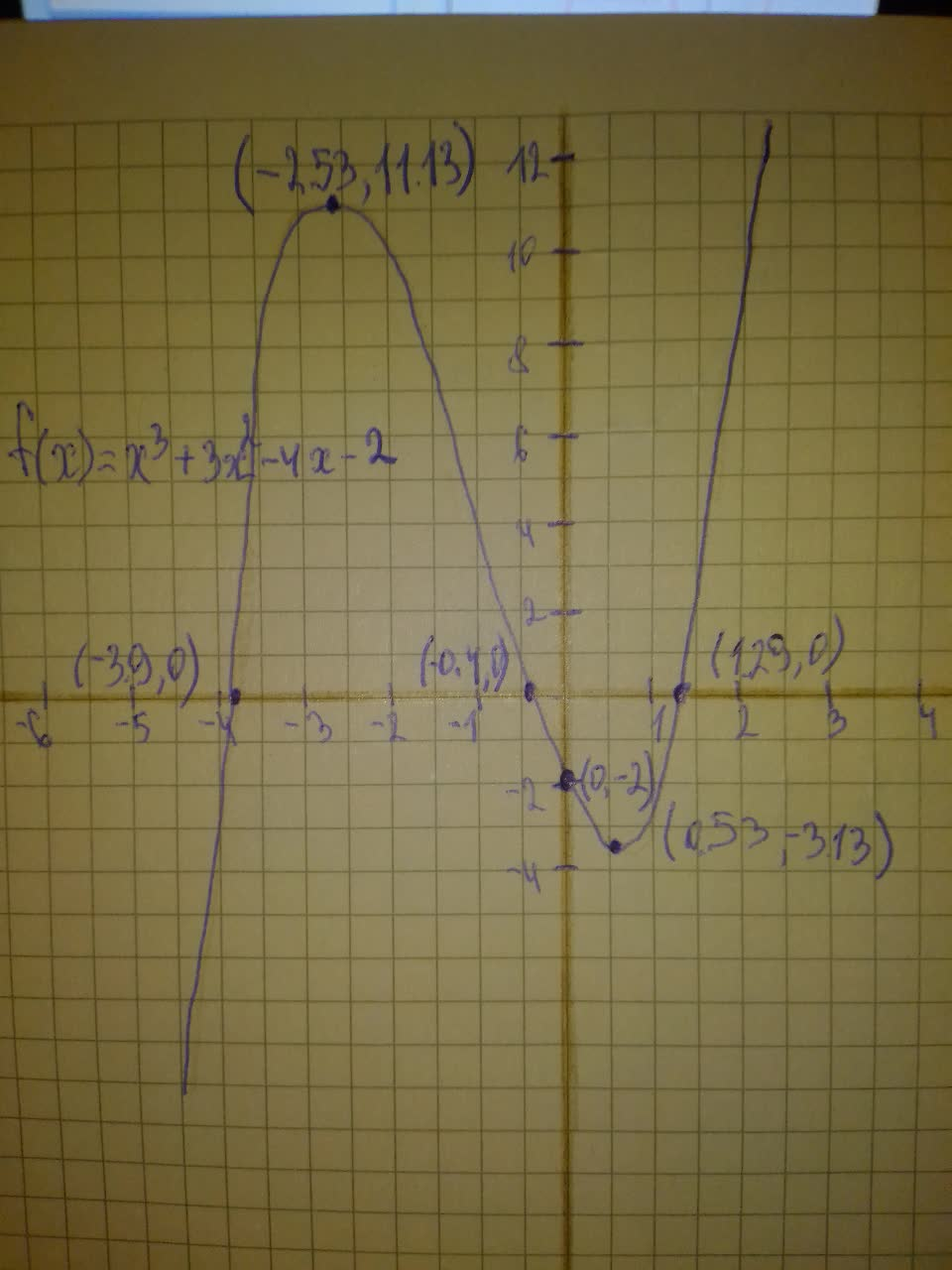Question# Graph each polynomial function. f(x)=x^{3}+3x^{2}-4x-2

Polynomial graphs
ANSWEREDGraph each polynomial function. $$\displaystyle{f{{\left({x}\right)}}}={x}^{{{3}}}+{3}{x}^{{{2}}}-{4}{x}-{2}$$2021-08-12
Step 1
Use a graphing calculator to graph
$$\displaystyle{f{{\left({x}\right)}}}={x}^{{{3}}}+{3}{x}^{{{2}}}-{4}{x}-{2}$$
The x-intercept are $$\displaystyle{\left(-{3.9},{0}\right)},{\left(-{0.4},{0}\right)}{\quad\text{and}\quad}{\left({1.29},{0}\right)}$$
The y -intercept is $$\displaystyle{\left({0},-{2}\right)}$$